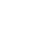# How to calculate Voltage Unbalance?

2
6846Voltage unbalance is a frequent problem in electrical power systems that can cause a variety of operational issues and even equipment damage. It describes the disparity between the three phases of a three-phase power system in terms of voltage magnitudes or phase angles. Maintaining electrical stability and guaranteeing optimal performance depends on accurately detecting and quantifying voltage imbalance.

This is the Voltage Unbalance Calculator as per NEMA MG-1- 2016. You can calculate voltage unbalance at the motor terminals.

## Voltage Unbalance Calculator

How to use the calculator?

• First note down the Line-Line voltage i.e., RY, YB, and BR at the motor terminal with the help of a multimeter.
• Enter those values in the calculator.

That’s it! Rest part calculator would do. Remember that as per NEMA MG-1-2016 the percentage voltage drop should not exceed more than 1% at the motor terminals.

## How to Calculate Voltage Unbalance?

There is simple math in Voltage Unbalance Calculation. What you have to do is –

1. Measure the line-to-line voltage (VRY, VYB, and VRB) across each pair of phases at the PCC level.
• Take out the average of line-to-line voltage (VLL_avg) by summing up the three line-to-line voltages and dividing the result by 3.

VLL_avg = (VRY+VYB+VRB) / 3

• Determine the maximum deviation of voltage (VD). Calculate the voltage deviation for each phase by subtracting the average line-to-line voltage from the respective line-to-line voltage,

VD_ry = VRY – VLL_avg,

VD_yb = VYB – VLL_avg,

VD_rb = VRB – VLL_avg.

Note down the maximum voltage deviation.

• Calculate the Voltage Unbalance in percentage using the below formula –

% Voltage Unbalance = (Maximum deviation from average voltage / Average Value) X 100

### Example for Calculating Voltage Unbalance

Example
Example: For example –

The measuring line voltages are 415, 411, and 410 volts.

The average voltage is (415+411+410 = 1236 / 3 = 412).

Voltage deviation for each phase is,

VD_ry = VRY – VLL_avg = 415-412 = 3

VD_yb = VYB – VLL_avg = 411-412 = |1|

VD_rb = VRB – VLL_avg = 410-412 = |2|

The maximum deviation from the average voltage is 3.

Putting the values in the formula,

%Voltage Unbalance = (3/412)*100

The voltage unbalance is 0.73%. So here, the voltage unbalance is within the limit as per NEMA Standard.

## Summarizing

If you are an Electrical professional you can provide a balanced and stable electrical power system, minimize the danger of expensive breakdowns, and assure optimal performance in varied industrial, commercial, and residential contexts by knowing how to calculate voltage imbalance.

Related Article

Also See other Calculators

1.Sethupathi
•Krunal Shah (Mod)